QuestionAnswers

# State the property of parallel lines used to divide a given segment to a given number of equal parts.

Hint: Draw a line segment of required length and construct two parallel lines with the help of a compass and by using the property to parallel lines make equal corresponding angles on given parallel lines. We can divide the segment into a given number of equal parts

Step 1:Lets start with the line segment which is to be needed to be divided into equal parts. Draw the line segment AB of the given length.Step 2: Now from A let’s draw a line segment forming an acute angle with AB and about the same length but it need not be exactly the same measurement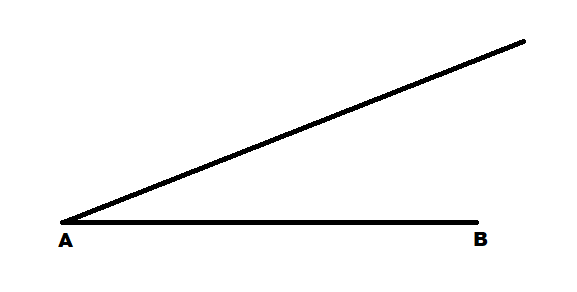Step 3:Now set the compass on A and mark the number of arcs required with equal measurement on the new line. The number of arcs required is the number of equal parts given in the problem.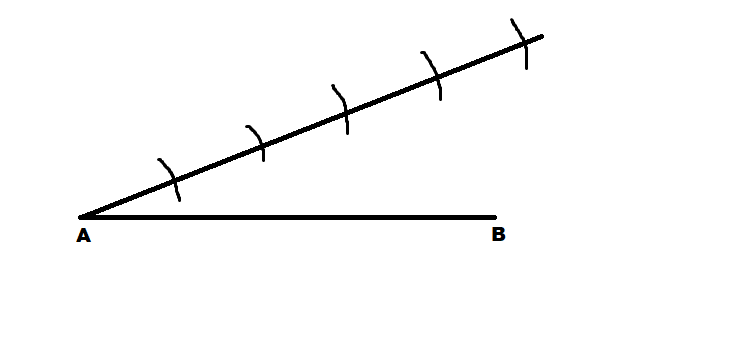Step 4: Now mark the intersection of the last arc with the line as C.
Measure the length of CB with the compass and with the same measurement lets draw an arc from A just below it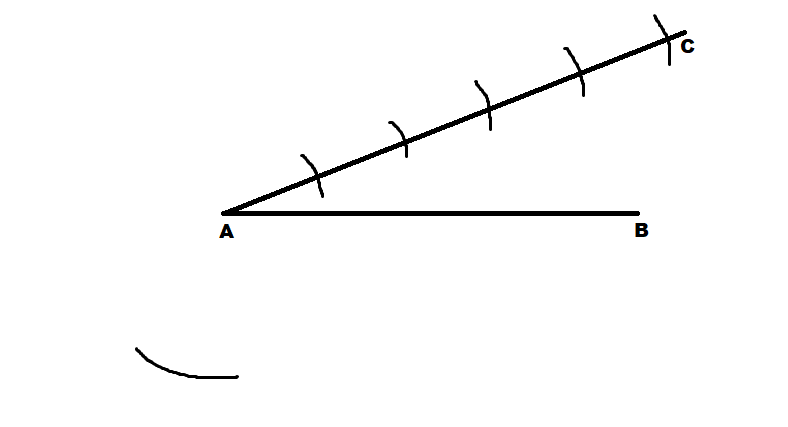Step 5: Now measure the length of AC with the compass and with the same measurement draw an arc from B crossing the arc drawn in our previous step.
Let that point be D and now join BD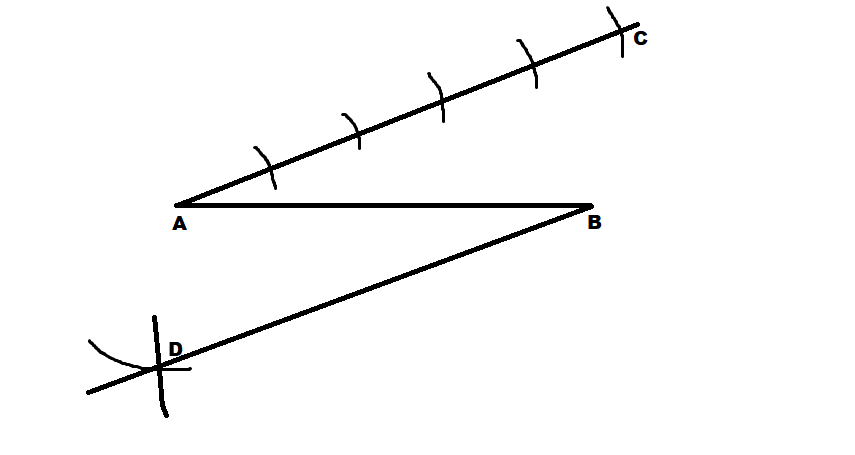Step 6: Now draw arcs on the line BD as we did in step 3 and with the same measurement.
But here we need to draw one arc less than the number we drew before.
Draw lines joining the corresponding points along with AC and BD
SS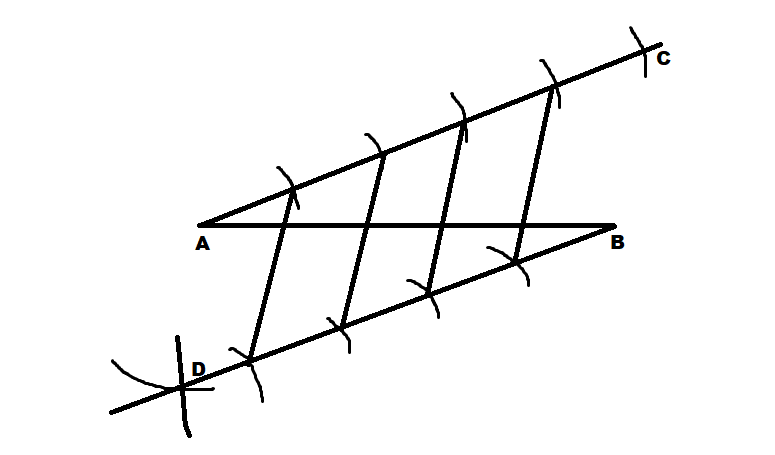Now the line segment is divided into equal parts.

Note: We can divide the segments into equal parts even by using a perpendicular bisector.
The properties used above are
When a transversal intersects a pair of parallel lines
(i)the corresponding angles are equal
(ii)the alternate interior angles are equal